(Class 9) Sum Of The Angles Of A Triangle Lesson Plan For B.Ed/DELED And School TeachersHello Friends, Welcome To Our Website. How Are You? Hope You Are Doing Well,

If You Are Searching For Sum Of The Angles Of A Triangle Lesson Plan Then You Have Come To The Right Place. Here We Have Shared The Lesson Plan On Sum Of Angle Of Triangle For B.Ed, D.El.Ed, And School Teachers.

This Mega Teaching Math Lesson Plan On Sum Of Angles Of A Triangle Is Specially Made For The B.Ed 1st And 2nd Year Students But All The Trainee Teacher And School Teachers Of All Classes Can Prepare Their Final Teaching Plan Very Easily With The Help Of This Model Sum Of The Angle Of A Triangle Lesson Plan For Class 8, 9, 10.

Brief Overview Of The Lesson Plan

 Class 9 Topic Sum Of Angle Of A Triangle Subject Mathematics Lesson Plan Type Mega Teaching Skill Real School Teaching And Practice

The Topics And Points That Are Covered In This Math Lesson Plan On Sum Of The Angles Of A Triangle Are : Triangle, Angle, Sum Of The Angle Of A Triangle, Question Answers And Examples…..

Sum Of The Angles Of A Triangle Lesson Plan –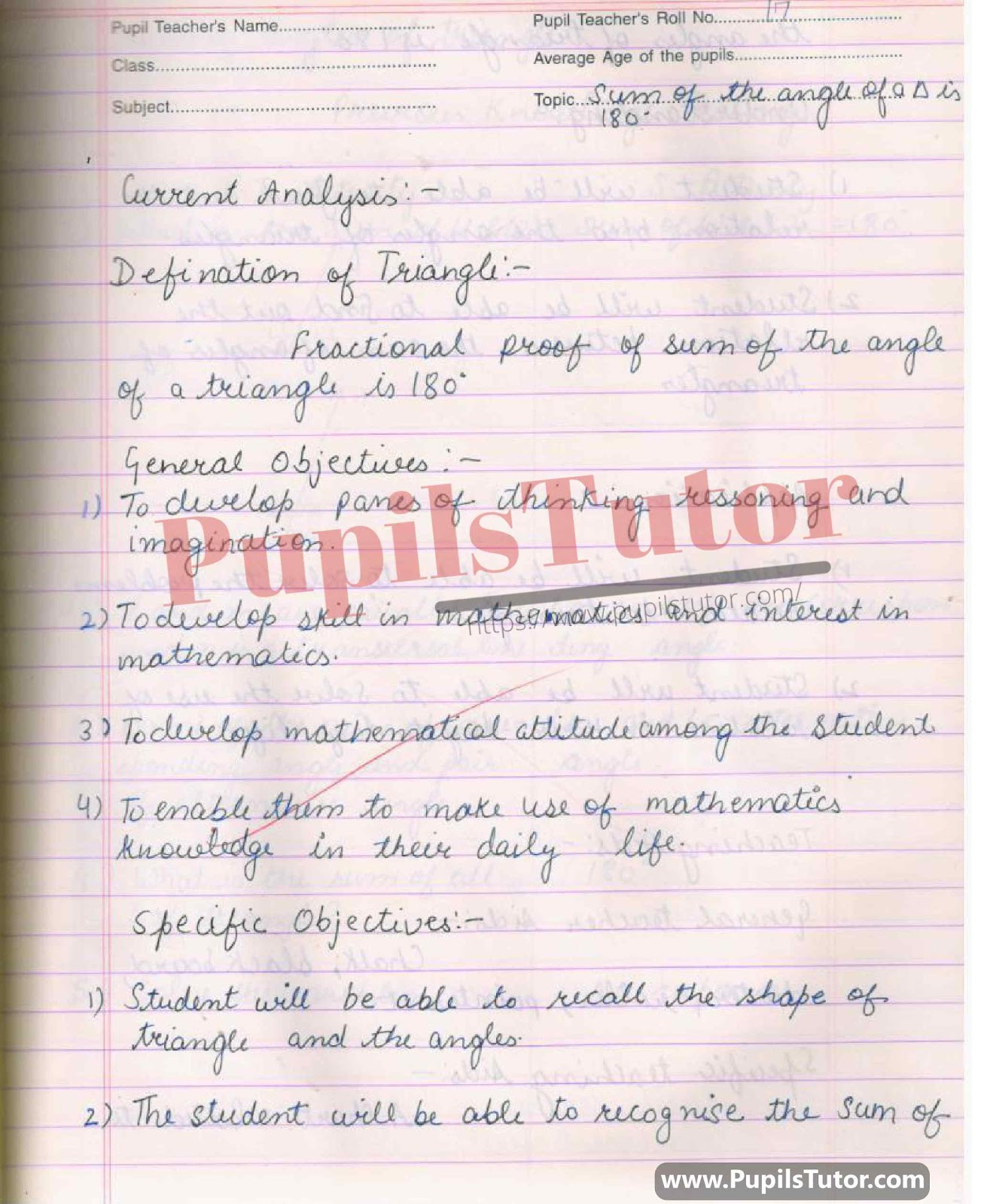Real And Mega Teaching Math Lesson Plan On Triangles, Sides, Angles, Sum Of The Angles Of A Triangle For B.Ed And Deled In English Free Download PDF And PPT (PowerPoint Presentation And Slides) –Maths Lesson Plan For Class/Grade 9 On Sum Of  Angles Of A Triangle Is 180 Degree, Triangle Diagram, Angle Theorem For CBSE NCERT School And College Teachers –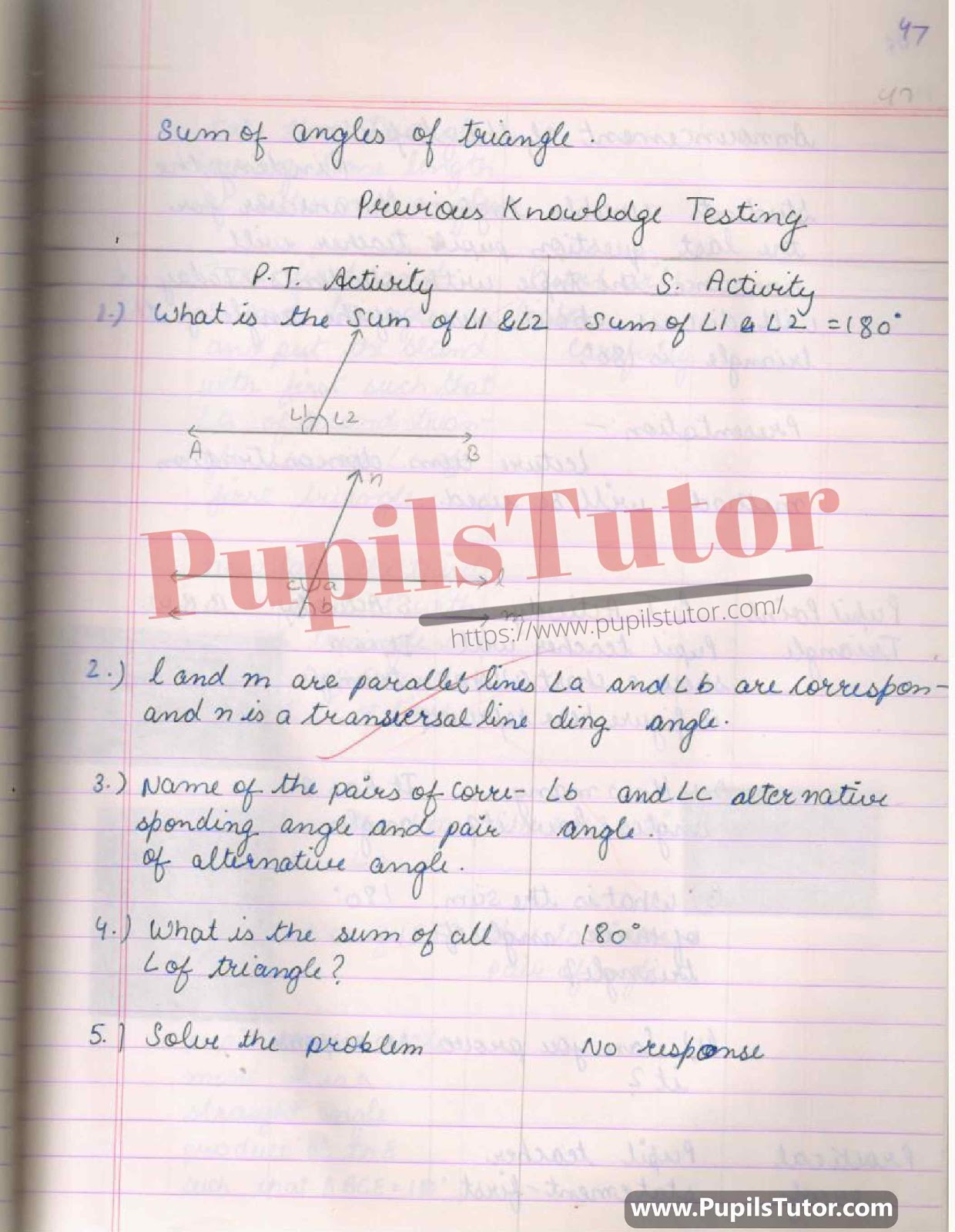BED, DELED, BTC, BSTC, M.ED, DED And NIOS Teaching Of Mathematics Innovative Digital Lesson Plan Format On Triangles, Sum Of The Angles Of A Triangle Topic For Class 7th 8th 9th, 10th–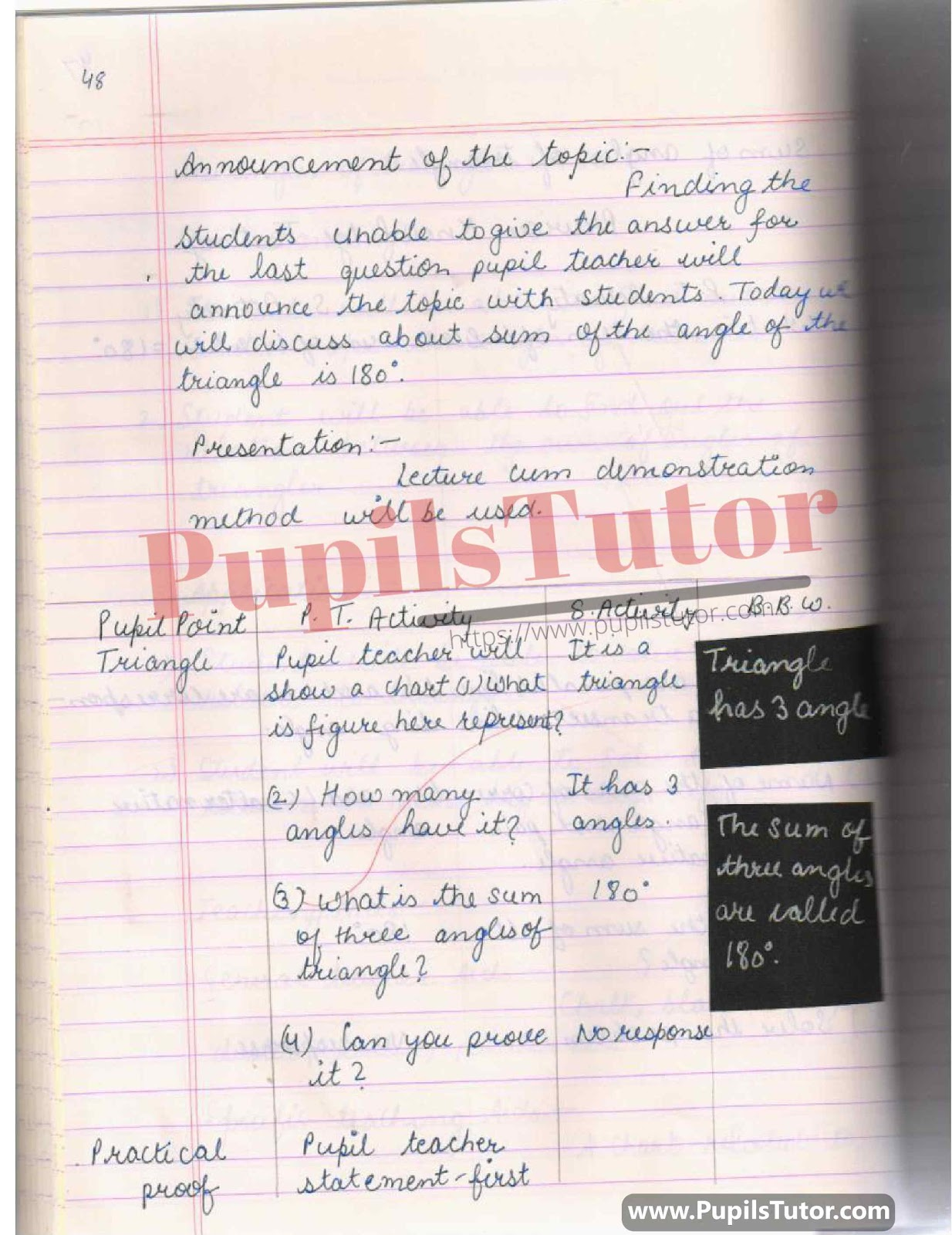Lesson Plan On Sum Of The Angles Of A Triangle With Latest Examples And Worksheet For Class 7th, 8th And 9th. –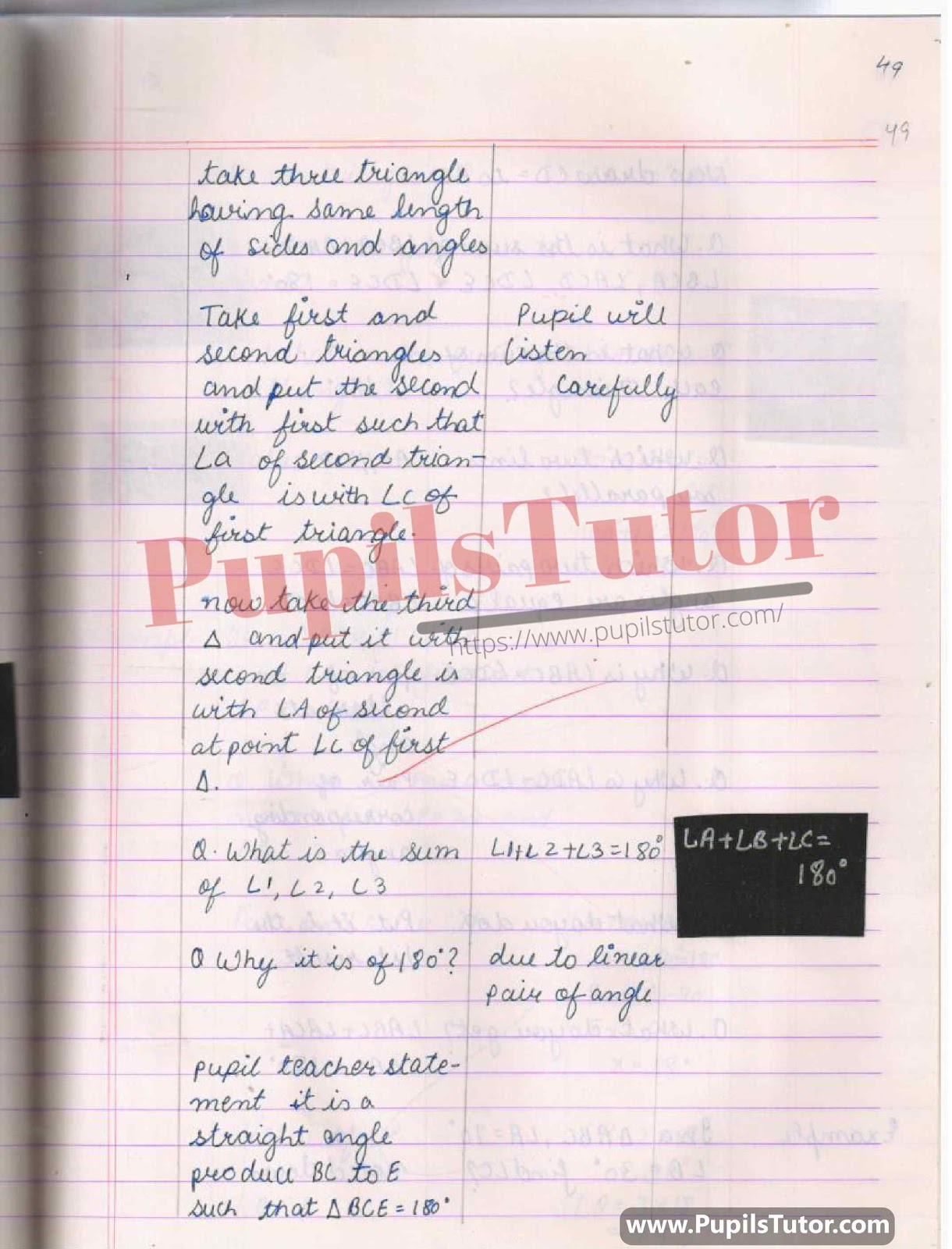Triangle Properties, Angle Theorem, Angle, Sum Of The Angles Of A Triangle Lesson Plan For B.Ed 1st Year, 2nd Year And All Semesters Students –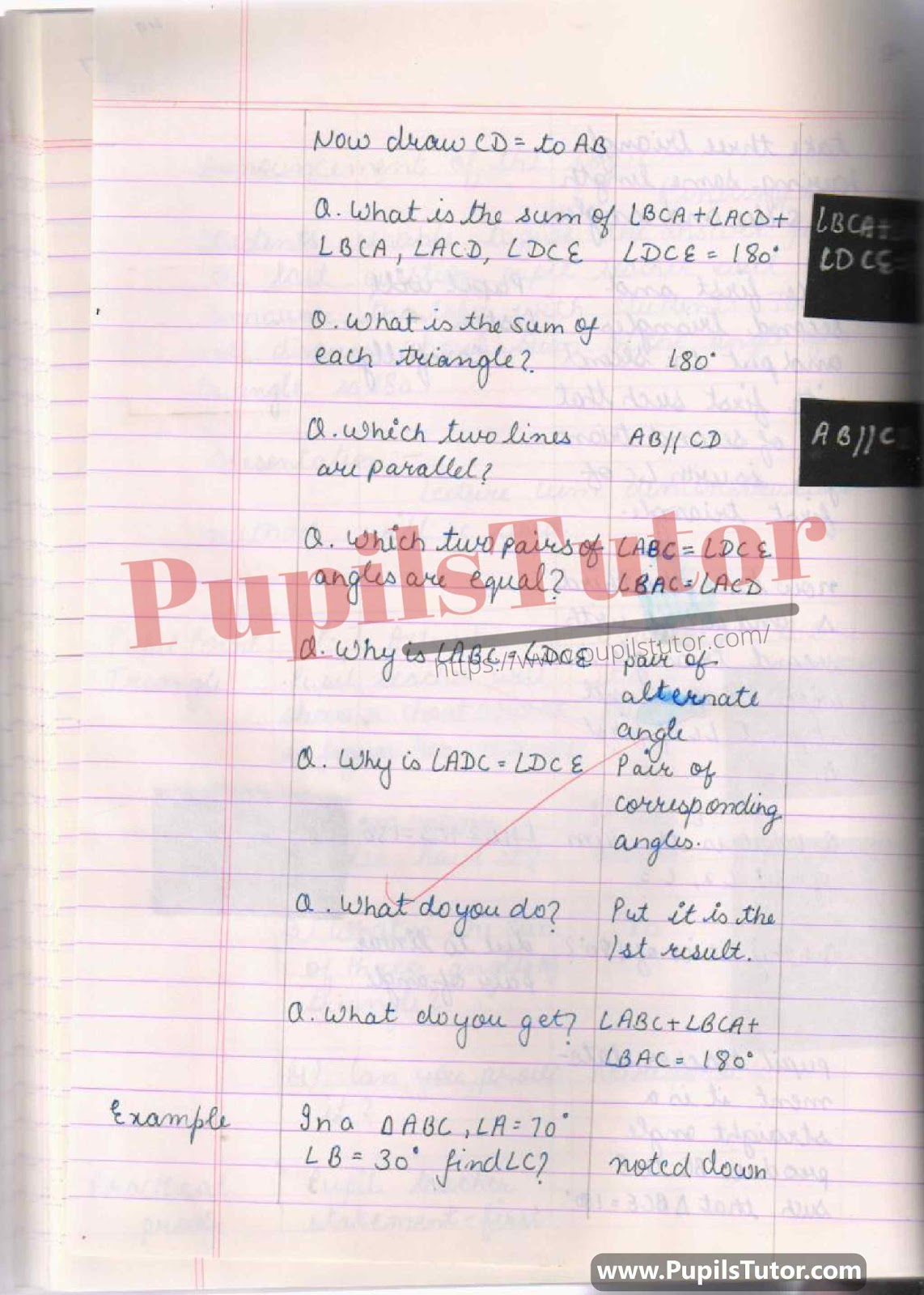How To Make Mega Teaching Sum Of The Angles Of A Triangle Lesson Plan For Math Subject In English –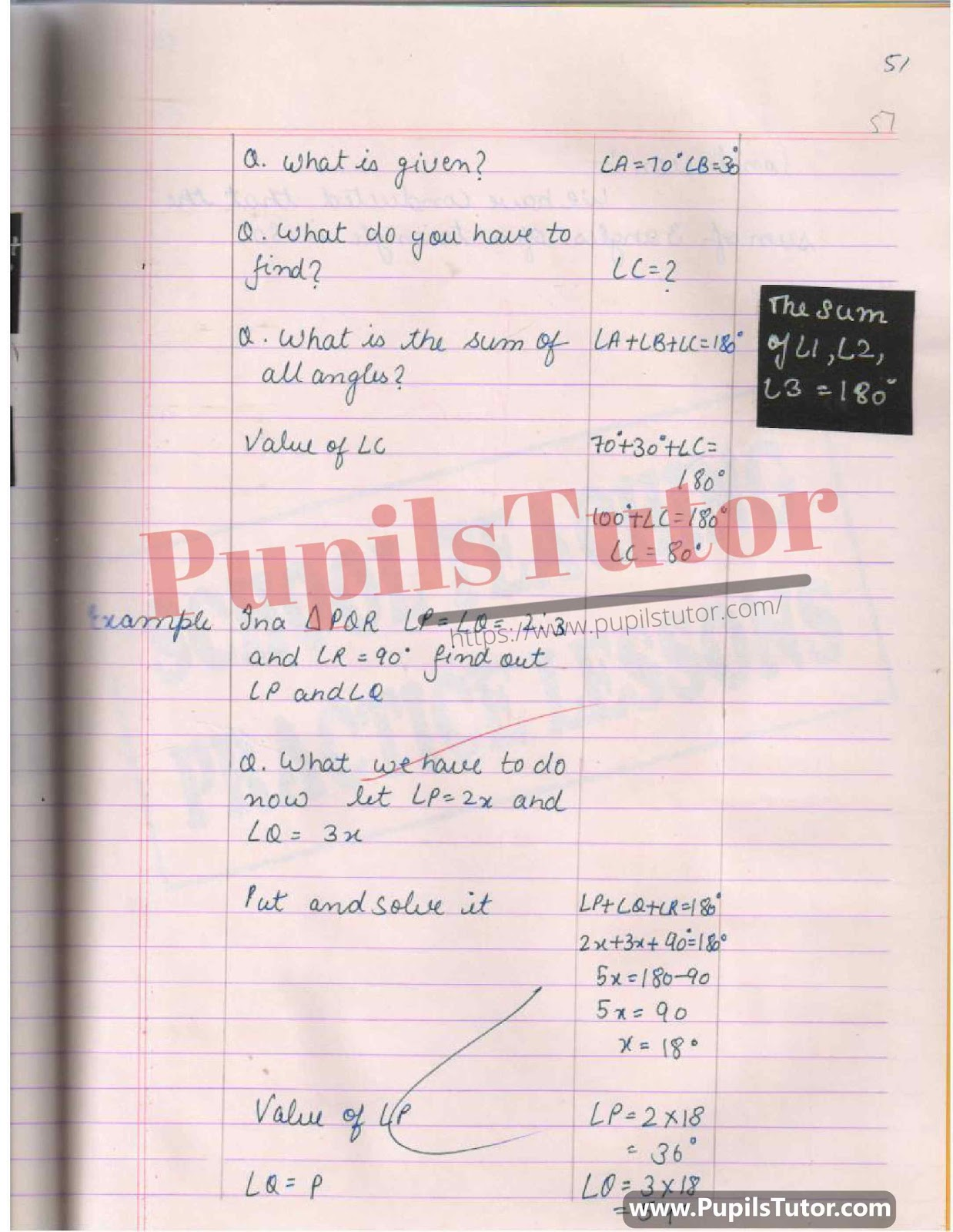Mathematics Lesson Plan On Triangle And Its Property, Proof Of Sum Of The Angles Of A Triangle Is 180 Degree Theorem PDF –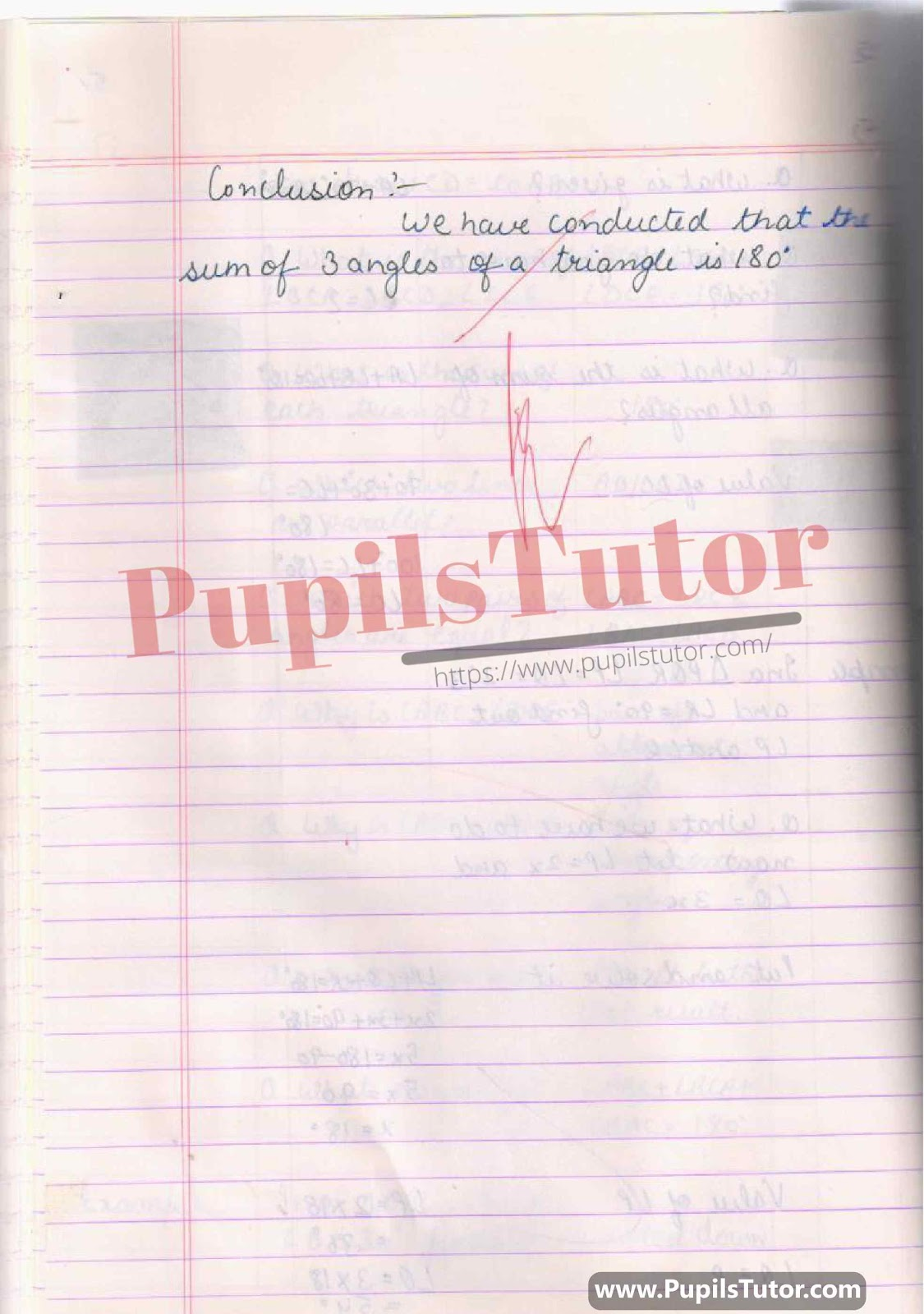On Our Website www.PupilsTutor.Com, We Have Also Shared Lots Of Other Lesson Plans Of All Subjects On Various Teaching Skills Like Micro-Teaching, Mega Teaching, Discussion Skill, Real School Teaching And Practice Skill, Simulated And Observation Skills. You Can Also Check Them.

If You Liked This Sum Of The Angles Of A Triangle Lesson Plan For Class 9 Then Please Share Our Efforts With Your Friends Also.

You Can Also Share Your Lesson Plans, Assignments, Files, Papers And Study Notes With Us To Help Other Students .